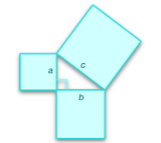# Ryangineer

## Machine Learning Mathematics&Virtual Reality Aesthetics

"In mathematics, you don't understand things; you just get used to them."
John von Neumann

### Precalculus Course Outline

#### Functions

• This section introduces the concept of functions, including function notation and evaluating functions.
•A diagram showing a function, such as f(x) = x^2, graphed on a Cartesian plane. Mathematics Libre Texts

#### Linear Functions

• Explore linear functions, including slope, intercepts, and graphing linear equations.
•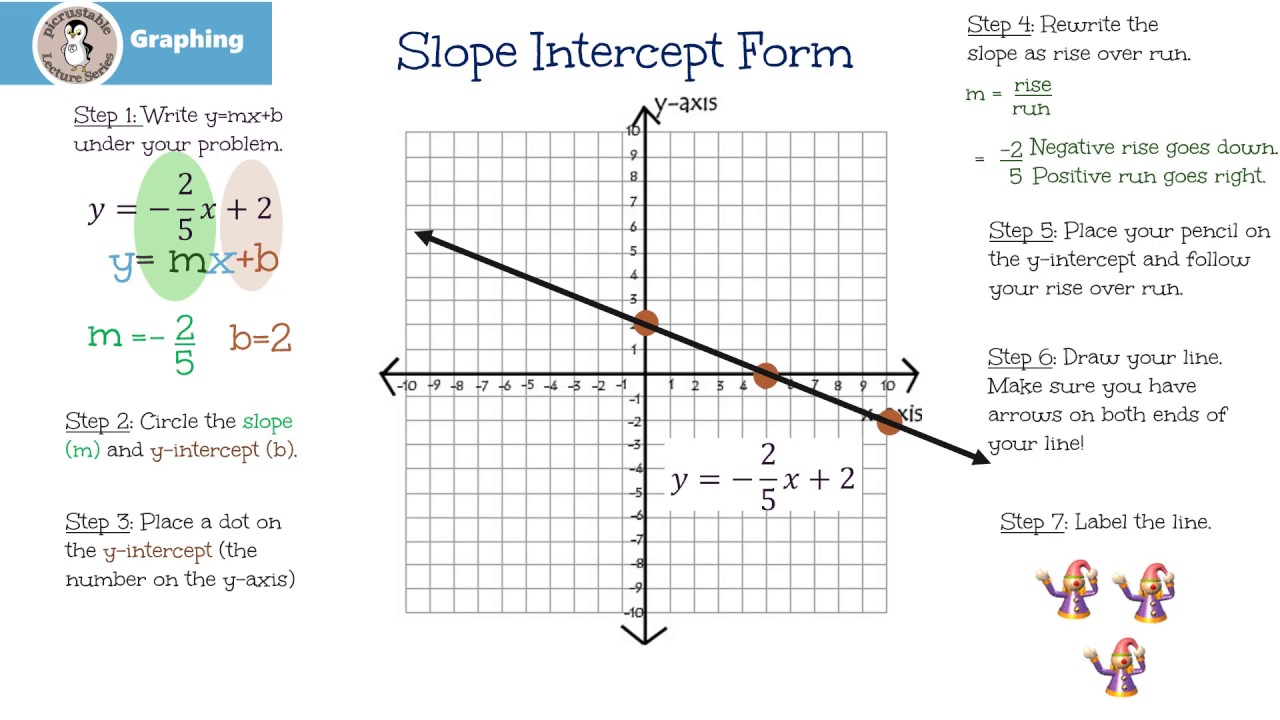Visuals illustrating the concept of slope and y-intercept, along with the graph of a linear equation, such as y = mx + b. School Tube

#### Systems of Linear Functions

• Learn about systems of linear equations and how to solve them using various methods such as substitution and elimination.
•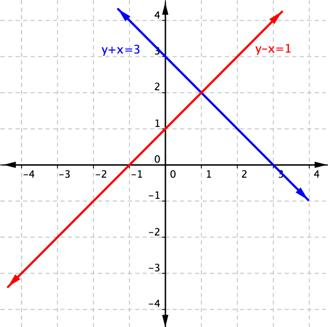An illustration of two linear functions graphed on the same plane showing their point of intersection. Open Textbook

#### Power and Polynomial Functions

• Study power functions and polynomial functions, including their properties and graphing techniques.
•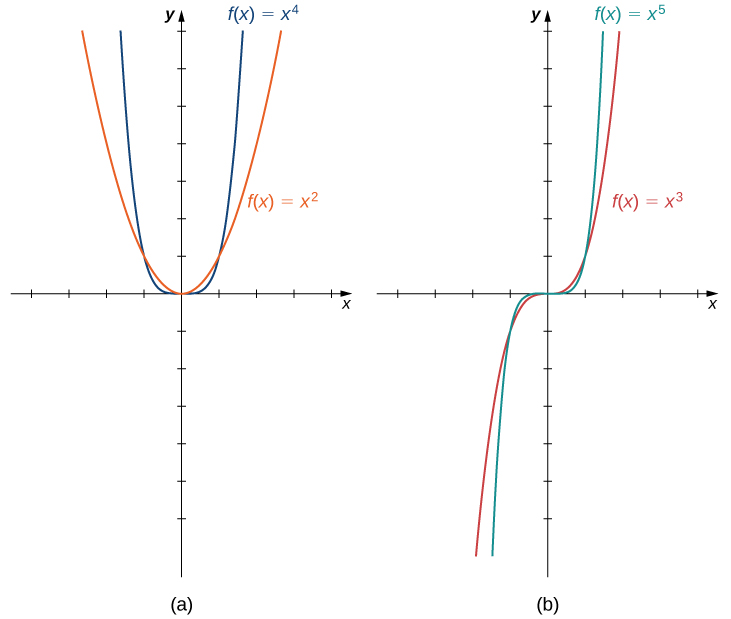Graphs of various polynomial functions, demonstrating different shapes depending on the degree of the polynomial. Lumen Learning

#### Rational Functions

• Examine rational functions and their characteristics, including asymptotes and solving rational equations.

#### Modeling Using Variation

• Understand the concept of variation and its applications in mathematical modeling.
•Diagrams showing direct, inverse, joint, or combined variation in real-world contexts. Slide Player

#### Exponential Functions

• Explore exponential functions, exponential growth and decay, and logarithmic properties.
•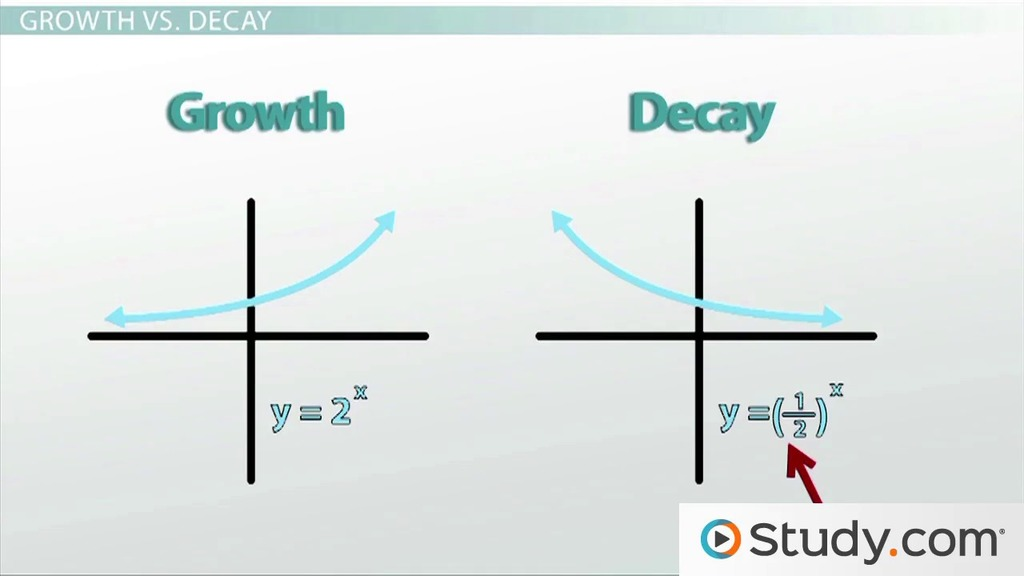A graph of an exponential function, showing the characteristic rapid growth or decay. Study.com

#### Logarithmic Functions

• Learn about logarithmic functions, logarithmic properties, and solving logarithmic equations.

#### Exponential and Logarithmic Equations and Models

• Apply exponential and logarithmic functions to solve equations and model real-life situations.
•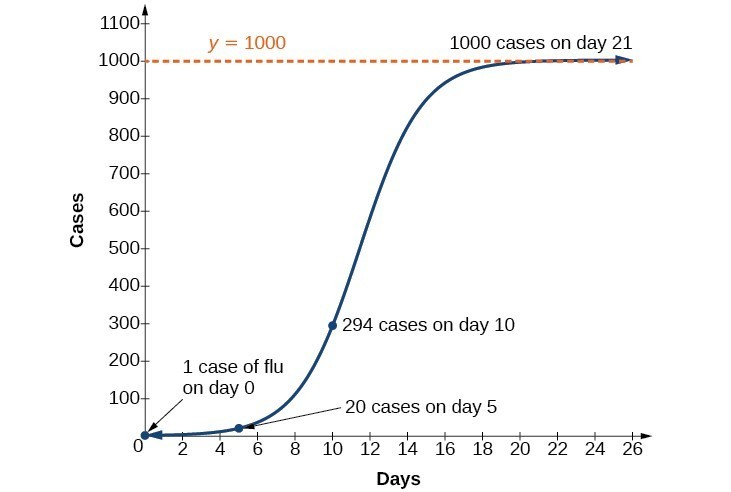Graphs depicting real-life situations that can be modeled with exponential or logarithmic functions, such as population growth or radioactive decay. Lumen Learning

#### Evaluating Trigonometric Functions

• Introduce trigonometric functions, including sine, cosine, and tangent, and learn to evaluate them.
•The unit circle diagram showing sine, cosine, and tangent values for different angles. Matter of Math

#### Graphing Trigonometric Functions

• Study the graphs of trigonometric functions and their transformations.

#### Trigonometric Identities

• Explore trigonometric identities, including Pythagorean identities and sum and difference identities.
•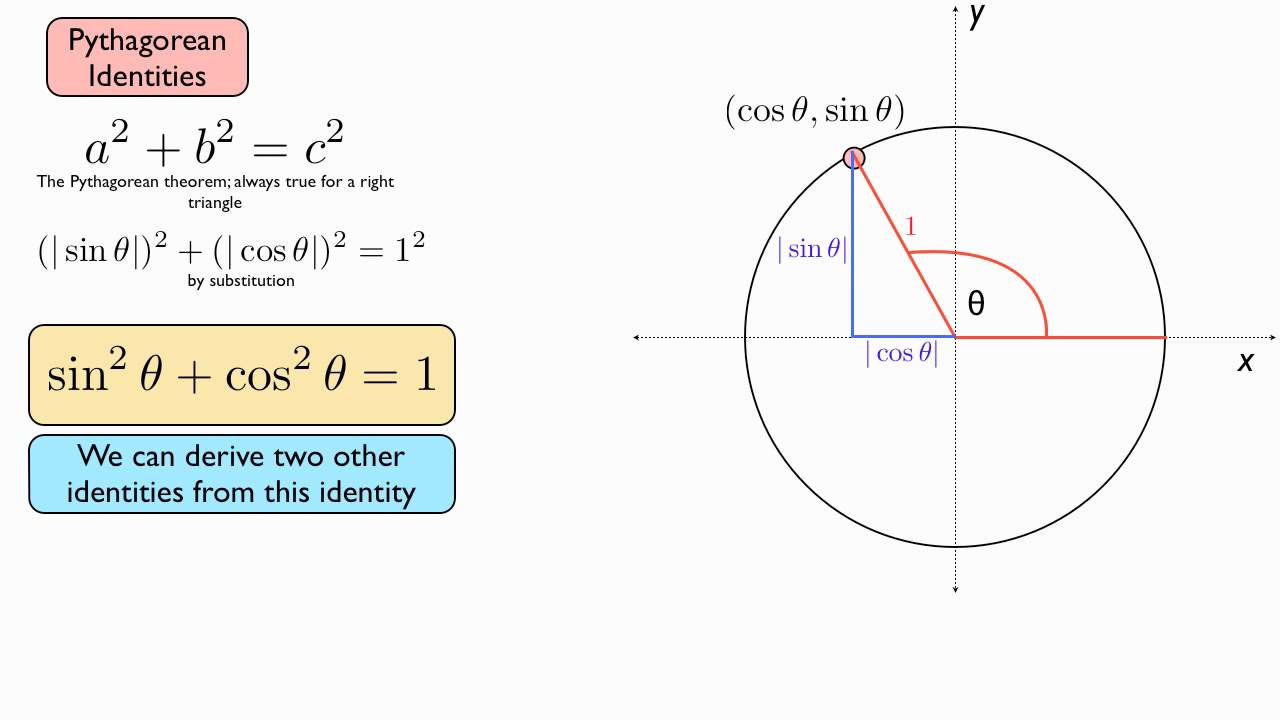Diagrams or proofs demonstrating trigonometric identities, such as those involving Pythagorean identities. Steve Stein

#### Inverse Trigonometric Functions and Solving Trigonometric Equations

• Understand inverse trigonometric functions and their properties, as well as solving trigonometric equations.
•Graphs of inverse trigonometric functions and illustrations of how they can be used to solve trigonometric equations. OnlineMathLearning.com

#### Further Applications of Trigonometry

• Apply trigonometric functions to solve real-world problems and explore additional trigonometric concepts.